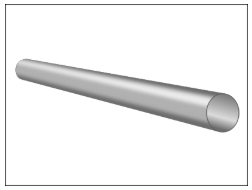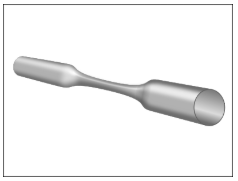# Current Electricity

Electricity is a set of physical phenomena associated with the presence of motion of electric charge. Electricity has various uses in everyday life. It is one of the easiest ways to convert energy. In the 1870s, incandescent light bulbs were invented. It was one of the first publicly available applications of electric power. In the 20th century, the invention of electronic devices changed the world of circuits. Transistors became the building blocks of modern circuitry. In modern integrated circuits, several transistors are placed in few square centimetres of a circuit.

Current

The movement of electric charge is known as current and is measured in amperes. Because electric charges are invisible, a hydraulic analogy with horizontal water flow is used to visualize the concepts. Electric current is equivalent to hydraulic volume flow rate, that is, the amount of water flowing over time.

Potential Difference

Potential difference between two points is the amount of work done by an electric charge to move from one point to another. It is measured in volts. It is equivalent to the pressure between two points in a pipe in the hydraulic analogy of horizontal water flow.

Resistance

A conducting wire is equivalent to a simple pipe (as shown in Figure 1) in hydraulic flow of horizontal water flow. The electric charge is equivalent to a quantity of water.Figure 1: Hydraulic Flow Analogy of a Conducting Wire

Resistors are electric components used to restrict the flow of charges. The resistance of a circuit element depends on its resistivity, length and cross-sectional area. A resistor is equivalent to a constriction in the pipe as shown in Figure 2. Thus, more pressure is required to pass the same amount of water. All pipes have some resistance to flow, just as all wires have some resistance to current.Figure 2: Hydraulic Flow Analogy of a Resistor

Conservation of Charge

The law of conservation of charge states that the total electric charge of an isolated system never charges.

Potential Divider

A potential divider is a circuit component realised with two resistors in series. It produces an output voltage that is a fraction of the input voltage. It is used to transform information from sensors like light-dependent resistor and thermistor into a quantity that can be used to display the information. The output of a potential divider is voltage.

Resistivity

The resistivity of a material is a fundamental property of a material that quantifies how strongly a given material opposes the flow of current. The resistivity of a material is highly dependent on temperature.

Current/ Voltage Characteristics

The relationship between current and voltage of various components will be analysed. Conductors for which I-V graphs are straight lines are said to be ohmic conductors. When the I-V graph is nonlinear, the conductor is said to be non-ohmic – a lamp and semiconductor diode are two examples of non-ohmic conductors.

Electromotive Force and Internal Resistance

The amount of energy supplied to a unit charge is defined as electromotive force (or EMF). In an SI unit system, the unit of EMF is volts. A cell is said to have an EMF of 1V when it supplies 1J of energy to each 1C of charge.

A source of EMF (for example, a battery) always has some resistance to electric current within it, called its internal resistance. It results in the voltage across the terminals of the battery dropping as a current is drawn from it.

Energy and Power in Circuits

The potential difference across a circuit element measures the energy transferred to it per unit charge flowing to it, the current measures the rate of flow of charge through it.

Potential difference is a measure of work done. Work is defined as the amount of energy transferred. The rate at which energy is transferred to a circuit element is called power dissipation. The unit for power is watts.

energy transferred (work done)=potential difference×charge

power=potential difference×current

When an electric current passes through a resistor, some of the electric energy is lost as heat. This is called Joule heating. The power dissipated is given by the following equation:

power=(current)^2×resistance